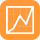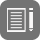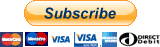## Roll Moment

by Racing Aspirations on April 10, 2020

New for 2020 are the roll center moment radius metrics which will allow you to calculate the roll center moment. The moment can be defined as the the tendency of a force to rotate an object around an axis. The moment radius is the distance between the roll center (axis) and the vehicle’s center of gravity (taken from the center of the chassis points for this basic calculation). It is difficult to keep this dimension the same across the range of the suspension travel, but keeping within a tolerance set by yourself is important.

The roll center is the point about which the suspension rotates (axis). If you imagine the line between the roll center and the center of gravity as a lever with your hand being the center of gravity then the longer that lever is the easier it is to rotate the suspension. Consider also that the weight of the vehicle is directly proportional to the force excerpted by you hand on that lever. This is why vehicle weight, the center of gravity, roll center location and roll center control are very important aspects of suspension design.

𝜏=𝐹𝑑

Moment = force x distance

The suspension geometry calculator gives you the distance from roll center moment radius (0.21m)

Force is the amount of force described as a weight that will push from the inside of a corner towards the outside. This is comparable to the your hand operating a lever and the amount of force you apply to the lever.

The sprung vehicle weight will also have an effect when calculating the force and you can use the weight distribution calculator to calculate the weight across a single axle if you so desire, but it is not essential for this simple example.

Using an online tool such as this or some simple math you can calculate the moment as follows:

moment = force * distance

moment = 100 kg * 0.21m

21 kg m = 100 kg * 0.21m

We can also express the same dimension as pounds and inches:

1823.2 lbs in = 220.46 lbs * 8.27 in

At the risk of over simplifying things, if you reduce either the force or the distance it reduces the moment which is a good thing as it mean the lateral force generated by cornering will have less effect on the roll of your vehicle. Great news!

## How can I see the moment radius?

1. Open the Macpherson Geometry Calculator or the Suspension Geometry Calculator
2. Clickto enable reports
3. Clickto open the report settings
4. Scroll down the list of metrics
1. Roll Center Moment Radius: The distance between the roll center and the center of gravity
2. Roll Center Moment Radius Offset: The difference between the static roll center moment radius (with no suspension movement) and the dynamic roll center moment radius (with suspension movement)
5. Click Apply to see the results

## What are the restrictions?to the Suspension Geometry Calculator |to the MacPherson Geometry Calculator